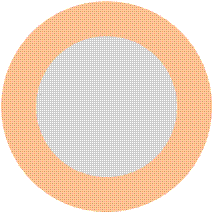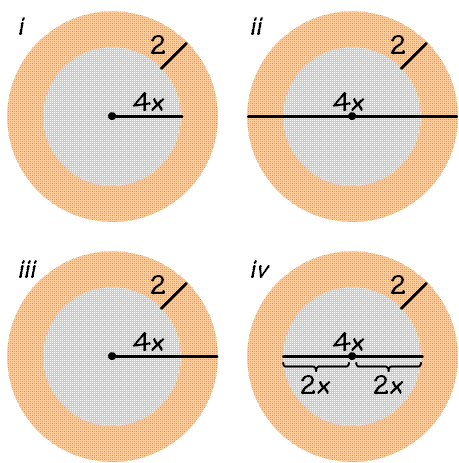# How to Draw Diagrams accurately for GMAT Geometry?

Drawing diagrams accurately in GMAT Geometry questions will allow you to capture the essential information from the Question. It’s not always easy. Here is a hands-on Experience for you: Draw the diagram as your read the question(below) and then go through the analysis.

Q) A circular table consists of a glass center surrounded by a metal ring of uniform width. If the metal ring has a width of 2 inches, and the glass center has a diameter of 4x inches, what fraction of the table’s surface is made up by the metal ring, in terms of x?

There’s no underhanded trick in this question, nor is there anything super complicated to incorporate into your diagram. But you should always be very mindful of the details of the question while drawing your diagram, since after you do so, you’re less likely to look at the information given in the problem. Indeed, it’s a waste of time to do so, since the information is presented much more usefully in your diagram! But this also means that if you make a mistake in the diagram, you may not correct it – and it’s very frustrating to get a problem wrong simply because your diagram was drawn incorrectly.

If it helps you to think figuratively, consider this metaphor I’ve *ahem* cooked up recently: If GMAT number properties are like soup, then GMAT geometry problems are like baking. Soup is a very forgiving dish: you can double the vegetables and halve the salt and the soup will still taste great. Same with number properties problems: for example, you can determine that the sum of an odd number and an even number is odd by memorizing that number property,  or you can just take a half second to plug in numbers (3 +2 = 5, 13 + -4 = 9, etc).

Baking, on the other hand, is a precise science: if you leave out the butter or salt, your cookies are going to fall flat. Same with GMAT geometry: you must take each step precisely, and there’s very little flexibility. When you draw a diagram, you must follow the “recipe” very precisely. Transferring information from text to diagram is a common place for careless mistakes to occur. Let’s look at the example problem again and draw the diagram step by step, keeping our eyes open for any places where mistakes might occur. Here is the problem again:

A circular table consists of a glass center surrounded by a metal ring of uniform width. If the metal ring has a width of 2 inches, and the glass center has a diameter of 4x inches, what fraction of the table’s surface is made up by the metal ring, in terms of x?

The first thing we are told is that the table consists of a circular glass center surrounded by a metal ring of uniform width. Thus, we have two concentric circles:Next, a diameter and a width are given. The 2 inch width is fairly straightforward to fit into the diagram. The width of the orange band can be referred to, but width would not be used to describe a dimension of the glass circle. With respect to the diameter, the words are also clear: the glass center has a diameter of 4x inches. But this is actually where the trouble starts. This question is only one out of 37; we are worried about how much time we spend on any one problem. So, maybe we draw 4x as a radius instead of a diameter (diagram i) , or as the diameter of the whole table instead of only the glass center (diagram ii), or as the radius of the whole table (diagram iii).The last diagram (iv) is of course correct. This isn’t complicated, but it is important to realize that these are the situations where we can slip and lose points needlessly.

And of course, once you draw the diagram, you still have to do the rest of the work. What did you get for this problem?

Knewton
was a leading test prep company that helped thousands of GMAT test takers since 2008. As of 2014, Knewton has discontinued offering tutoring service.

Recommended GMAT Prep Resources

Mastering GMAT Critical Reasoning1. Complete GMAT RC Questions in less than 1 minute and 50 seconds
3. Take Notes Effectively
4. Collect and Interpret Facts
5. Speed up Summary Creation
6. Remember Information
7. Question the Author

12. Learn to Answer GMAT organization of passage Question
13. Learn to identify the style/tone or attitude of the author

Mastering GMAT Critical ReasoningAfter you read F1GMAT’s Mastering GMAT Critical Reasoning Guide, you will learn:

How to overcome flawed thinking in GMAT Critical Reasoning?

How to spot Inconsistencies in Arguments

How to eliminate out of scope answer choices using Necessary and Sufficient Conditions

How to Paraphrase GMAT Critical Reasoning Question

How to Answer Assumption Question Type

How to Answer Conclusion Question Type

How to Answer Inference Question Type

How to Answer Strengthen Question Type

How to Answer Weaken Question Type

How to Answer bold-faced and Summary Question Types

How to Answer Parallel Reasoning Questions

How to Answer the Fill in the Blanks Question

Get F1GMAT's Newsletters (Best in the Industry)

• Ranking Analysis
• Post-MBA Salary Trends
• Post-MBA Job Function & Industry Analysis
• Post-MBA City Review
• MBA Application Essay Tips
• School Specific Essay Tips
• GMAT Preparation Tips